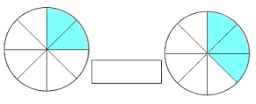# One-sixth 14133

Subtract one-sixth of this unknown number from three times the unknown number, and you get the number 17. Calculate the unknown number

x =  6

### Step-by-step explanation:

3x-x/6=17

3·x-x/6=17

17x = 102

x = 102/17 = 6

x = 6

Our simple equation calculator calculates it.Did you find an error or inaccuracy? Feel free to write us. Thank you!

Tips for related online calculators
Need help calculating sum, simplifying, or multiplying fractions? Try our fraction calculator.
Do you have a linear equation or system of equations and looking for its solution? Or do you have a quadratic equation?